Next: Transfer orbits Up: Keplerian orbits Previous: Orbital parameters

# Orbital energies

Let us now generalize our analysis to take into account the orbits of asteroids and comets about the Sun. Such orbits satisfy Equation (4.30) but can be parabolic (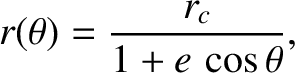) or even hyperbolic (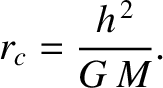), as well as elliptical (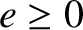). According to Equations (4.5) and (4.13), the total energy per unit mass of an object in a general orbit around the Sun is given by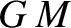(4.41)

It follows from Equations (4.22), (4.26), and (4.31) that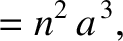(4.42)

where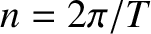, and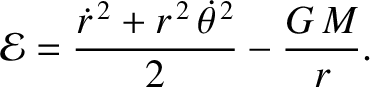. However, according to Equation (4.30),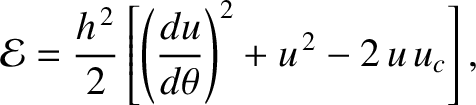(4.43)

The previous two equations can be combined with Equations (4.31) and (4.34) to give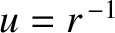(4.44)

We conclude that elliptical orbits () have negative total energies, whereas parabolic orbits () have zero total energies, and hyperbolic orbits () have positive total energies. This makes sense because in a conservative system in which the potential energy at infinity is set to zero [see Equation (4.4)], we expect bounded orbits to have negative total energies, and unbounded orbits to have positive total energies. (See Section 2.7.) Thus, elliptical orbits, which are clearly bounded, should indeed have negative total energies, whereas hyperbolic orbits, which are clearly unbounded, should indeed have positive total energies. Parabolic orbits are marginally bounded (i.e., an object executing a parabolic orbit only just manages to escape from the Sun's gravitational field), and thus have zero total energy. For the special case of an elliptical orbit, whose major radiusis finite, we can write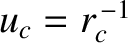(4.45)

It follows that the energy of such an orbit is completely determined by its major radius.Next: Transfer orbits Up: Keplerian orbits Previous: Orbital parameters
Richard Fitzpatrick 2016-03-31